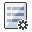Expert Modelling: Hands-on help from Rocscience Engineers. Register Here

# Forces

If you are performing a Probabilistic analysis with SWedge, the following forces can be defined as random variables:

NOTE: It is not possible to define External forces as random variables.

## Water Pressure (Probabilistic)

To define Ponded Water Pressure as a random variable in a probabilistic analysis:

1. Select Input Dataand select the Ponded Water tab.
2. Select the Ponded Water Pressure check box.
You can define the Ponded Water Depth as a random variable.
3. NOTE: Joint Water Pressure Distribution Model is unavailable when the Pervious option is selected.

To define Joint Water Pressure as a random variable in a probabilistic analysis:

4. Select Input Dataand select the Water tab.
5. Select the Joint Water Pressure check box.
6. Joint Water Pressure can be defined as a random variable if you are using the % Filled Fissures option or the Custom Pressure option.
• If the Type = % Filled Fissures slope type or Slope Face Type = Pervious, you can define the Percent Filled as a random variable
• If the Type = Custom Pressure, you can define the Joint1 Pressure and/or the Joint2 Pressure as random variable(s).

For details about the Water Pressure options see the Water Pressure topic.

## Seismic Force (Probabilistic)

To define Seismic Force as a random variable in a probabilistic analysis:

1. Select Input Dataand select the Seismic tab.
2. Select the Seismic check box.
• You can define the Seismic Coefficient as a random variable
• If the Direction = User Defined, you can define the Plunge and/or Trend as a random variable.

For details about the Seismic Force options see the Seismic Force topic.

## External Force (Probabilistic)

At present it is NOT possible to define an External force (i.e. point load) as a random variable in an SWedge probabilistic analysis. You can include constant External Force(s) in a probabilistic analysis, but you cannot define the force as a random variable.

For details about the External Force option see the External Force topic.